# Wood cuboid

What is the weight of the wood cuboid 15 cm, 20 cm, 3 m if 1 m3 wood weighs 800 kg?

Result

m =  72 kg

#### Solution:Leave us a comment of example and its solution (i.e. if it is still somewhat unclear...):

Showing 0 comments:Be the first to comment!#### To solve this example are needed these knowledge from mathematics:

Do you want to convert length units? Tip: Our volume units converter will help you with converion of volume units.

## Next similar examples:

1. Cuboid 5Calculate the weight of the cuboid with dimensions of 12 cm; 0.8 dm and 100 mm made from spruce wood (ς = 550 kg/m3).
2. Glass doorWhat is the weight of glass door panel 5 mm thick height 2.1 meters and a width of 65 cm and 1 cubic dm of glass weighs 2.5 kg?
3. Air massWhat is the weight of the air in a classroom with dimensions 10 m × 10 m × 2.7 m ? The air density is 1.293 kg/m3.
4. Fe vs. H2O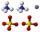The volume of what the body of the same weight is greater - iron or water?
5. Weight of airWhat is the weight of air in the living room measuring width 5 m length 2 m and height 2.8 m? Air density is ρ = 1.2 kg/m3.
6. Stone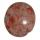When Peter threw stone in a box of water he discovered that the water level has risen by 9 cm. The box has a cuboid shape, the bottom has dimensions of 24 cm and 14 cm, height is 50 cm. What volume has a stone?
7. Swimming pool 4The pool shaped cuboid measuring 12.5 m × 640 cm at the bottom is 960hl water. To what height in meters reaches the water level?
8. Water poolWhat water level is in the pool shaped cuboid with bottom dimensions of 25 m and 10 meters, when is 3750hl water in the pool.
9. Oak cuboidOak timber is rectangular shaped with dimensions of 2m, 30 cm and 15 cm. It weight is 70 kg. Calculate the weight 1 dm³ of timber.
10. Cuprum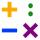From 1600 mm long sheet of copper plate 2 mm thickness we have separated over the whole length the belt weighing 6000 g. Calculate belt width if 1 dm3 copper weighs 8.9 kg.
11. Fire tankHow deep is the fire tank with the dimensions of the bottom 7m and 12m, when filled with 420 m3 of water?
12. Concrete boxThe concrete box with walls thick 5 cm has the following external dimensions: length 1.4 m, width 38 cm and height 42 cm. How many liters of soil can fit if I fill it to the brim?
13. Cuboid - simple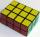Calculate the surface area and volume of a cuboid if a = 8 cm, b = 14 cm and c = 6 cm.
14. Cuboid aquariumCuboid 25 times 30 cm. How long is third side if cuboid contains 30 liters of water?
15. Metal pyramid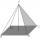Find the weight of a regular quadrilateral pyramid with a 5 cm length and 6,5 cm body height is made from material with density g/cm3.
16. Solid in waterThe solid weighs in air 11.8 g and in water 10 g. Calculate the density of the solid.
17. Excavator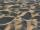The excavator bucket capacity is 0.5 m3. Determine the mass of sand that the excavator picks up. The sand density is 1650 kg/m3.